# Complete Guide To Linked Lists

Linked list is a linear data structure that means it is traversed in a specific sequence or order.
In simple words it is a list consisting of items in which each item knows the location of the next item.
A piece of list data and the location of the next item (node) is contained in an item called node. A pointer in each node determines the order of a linked list. It is only the address in memory of the next node. A linked list can be visualized as a chain of nodes where each node contains the location of the next node.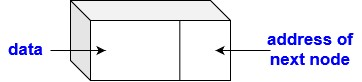Structure of a node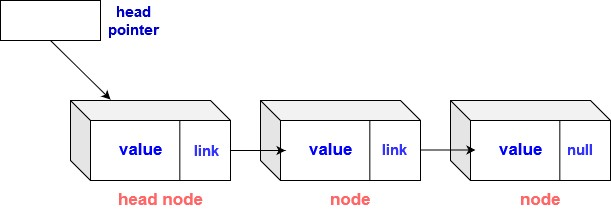Linked List

### Terminology

Head pointer: Points to the head node.

Head node: It is the first node of the linked list. Different operations can be performed on the linked list through head node. The reference of the head node of the linked list is generally known in problems.

Link: (of a node) is the location or address of the next node.

Null: The last node of the linked list points to null (none) which indicates that it is the last node.

## Array vs Linked Lists

• Unlike arrays, elements in a linked list are not stored at contiguous memory locations.
• In an array, memory is assigned during compile time while in a Linked list, memory allocation occurs during execution or runtime.

• Linked Lists addresses some of the limitations of arrays of having a fixed size because Linked Lists are dynamic in nature.This dynamic nature allows memory allocation whenever required.

## Types of linked lists

Following are the types of linked list:

1. Singly Linked List.
2. Doubly Linked List.
3. Circular Linked List.

### Singly Linked Lists

In a singly linked list, the node consists of one data field and one address field.Structure of a nodeLinked List

##### Representation of a node:
``````class Node {
int data // stores the data of the node
Node next//stores address of the next node
}
``````
##### Creating a linked list of 3 elements :
``````node *head;
node *n1 = NULL;
node *n2 = NULL;
node *n3 = NULL;

//Allocating memory
n1 = new Node();
n2 = new Node();
n3 = new Node();

// Assigning data values
n1->data = 1;
n2->data = 2;
n3->data = 3;

//Connecting nodes
n1->next = two;
n2->next = three;
n3->next = NULL;

//Saving address of first node in head
head = n1;

``````

### Doubly Linked Lists

A doubly linked list is a data structure in which each node contains one data link and two link fields. Thus there is a pointer to the next as well as the previous node in a sequence.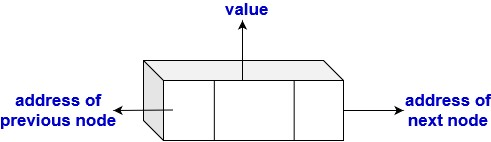node in a doubly linked list

##### Representation of node:
``````struct node
{
int data;
node next; //reference to the next node in a sequence
node prev; //reference to the previous node in a sequence
}

``````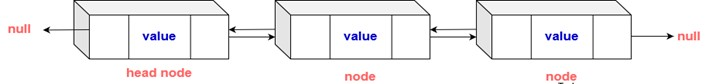doubly linked list

##### Creating a linked list of 3 elements :
``````node *head;
node *n1 = NULL;
node *n2 = NULL;
node *n3 = NULL;

// Allocating memory
n1 = new node();
n2 = new node();
n3 = new node();

// Assigning data values
n1->data = 1;
n2->data = 2;
n3->data = 3;

// Connecting nodes
n1->next = n2;
n1->prev = NULL;

n2->next = n3;
n2->prev = n1;

n3->next = NULL;
n3->prev = n2;

// Saving address of first node in head
head = n1;
``````

### Circular Linked List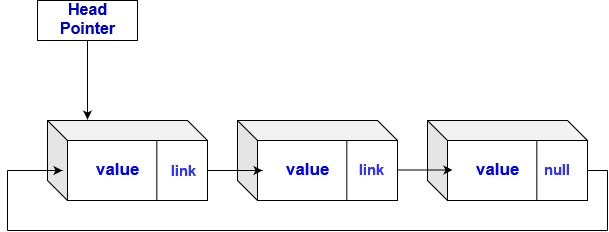It is a linked list which has no null values. It can be traversed from any node and in any direction.
The pointer in the last node contains the address of the first node of the list.
In a circular linked list, there is a need to define both the head and the last(or end) node.

Structure of a circular linked list

`````` struct Node
{
int data;
node next;
};
node* head = NULL;// defining first and last node of the list
node* end = NULL;
``````

## Operations on Linked lists

### 1. Traversing a linked list

To print the list or search for a specific node in the list, we need to go through the entire list from beginning to end.

Steps

• Start with the head of the list. Access the content of the head node if it is not null.

• Go to the next node if it exists and access its information.

• Continue till the null node is reached.

Code

``````void traverse(Node* head)
{
Node* temp = head;
while(temp != NULL)
{
print(temp.data)
temp = temp.next
}
}
``````

### 2. Insertion in a linked list

2.1. Insertion from front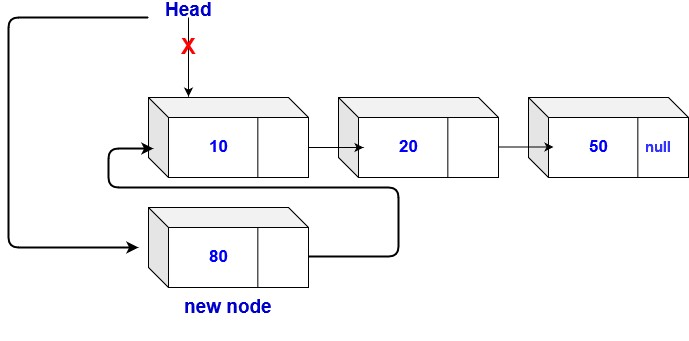Steps

• Finding the end of the list is not required here

• If the list is empty, a new node is made as the head of the list.

• Otherwise, the new node is connected to the current head of the list.

• The new node is made the head of the list.

Code

``````// returns the head of the singly linked-list
Node insertFront(Node head, int x)
{
temp = new Node(x)//creating new node

if(head == NULL) // check if linked list is empty
return temp
else // insert the node at the beginning
{
temp.next = head
return temp
}
}
``````

2.2. Insertion at the end: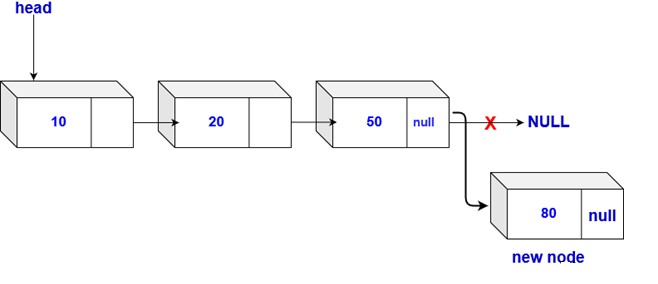Steps

• Traverse the list until last node is found.
• Insert the new node to the end of the list.
• If the list is empty, return the updated head of the linked list since the inserted node is the first as well as the last node of the linked list. (only one element)

Code

``````Node insertEnd(Node head, int x)
{
if( head == NULL )//condition for empty list
{
newNode = new Node(x)
head = newNode
return head
}
Node temp = head
// traversing the list to get the last node
while( temp.next != NULL )
{
temp = temp.next
}
newNode = new Node(x)
temp.next = newNode
return head
}
``````

2.3.Insertion at the nth position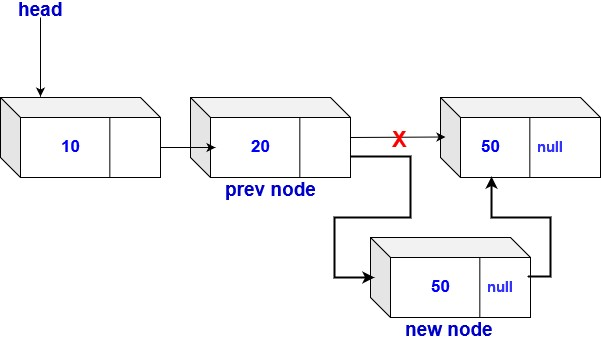Steps

• The reference to a node is given, and the new node is inserted after the given node.
• If the address of the prevNode is not given, then it can be found by traversing to that node by finding the data value.

Code

``````void Insert(Node prevNode, int x)
{
newNode = new Node(x)

newNode.next = prevNode.next
prevNode.next = newNode
}
``````

### 3.Deletion in linked list: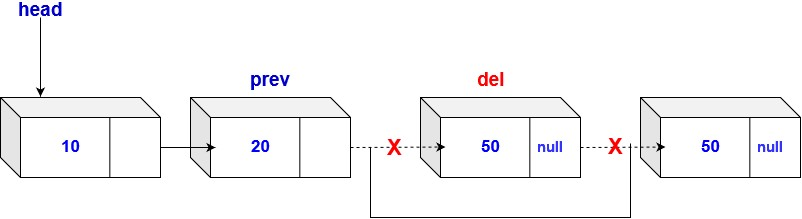Steps
To delete a node from a linked list, the following steps are to be followed:

• Find the previous node of the node to be deleted.
• Change the next pointer of the previous node
• Free the memory of the deleted node.
In the deletion, there is a special case in which the first node is deleted. In this, the head of the linked list must be updated.

Code

``````Node deletion(Node head, Node del)
{
if(head == del)//if headnode is to be deleted
{
return head.next // special case for the first Node
}
Node temp = head

while( temp.next != NULL )
{
if(temp.next == del) // finding the node to be deleted
{
temp.next = temp.next.next
delete del // freeing the memory of that Node
return head
}
temp = temp.next
}
return head// if node not found
}
``````

### 4. Searching

Code

``````bool search(Node head, int x)
{
Node temp = head // creating a temp variable which points to the head

while( temp != NULL) // traversing
{
if( temp.data == x )
return true
temp = temp.next
}
return false
}
``````

### 5. Updating a node

To update the value of the node, set the data part equal to the new value.

Code

``````void update(Node head, int x, int newx)
{
Node temp = head
while(temp != NULL)
{
if( temp.data == x)
{
temp.data = newx
return
}
temp = temp.next
}
``````

## Time Complexities

#### Singly Linked list

Operation Time Complexity
Insert from front O(1)
Insert from back O(n)
Insert after nth position O(1)
Insert before nth position O(n)
Deletion O(n)
Find Element O(n)

#### Doubly Linked list

Operation Time Complexity
Insert from front O(1)
Insert from back O(n)
Insert after nth position O(1)
Insert before nth position O(1)
Deletion O(n)
Find Element O(n)
Scroll to Top

### Full Stack Integrated Bootcamp Free Trial

• Please enter a number from 7000000000 to 9999999999.